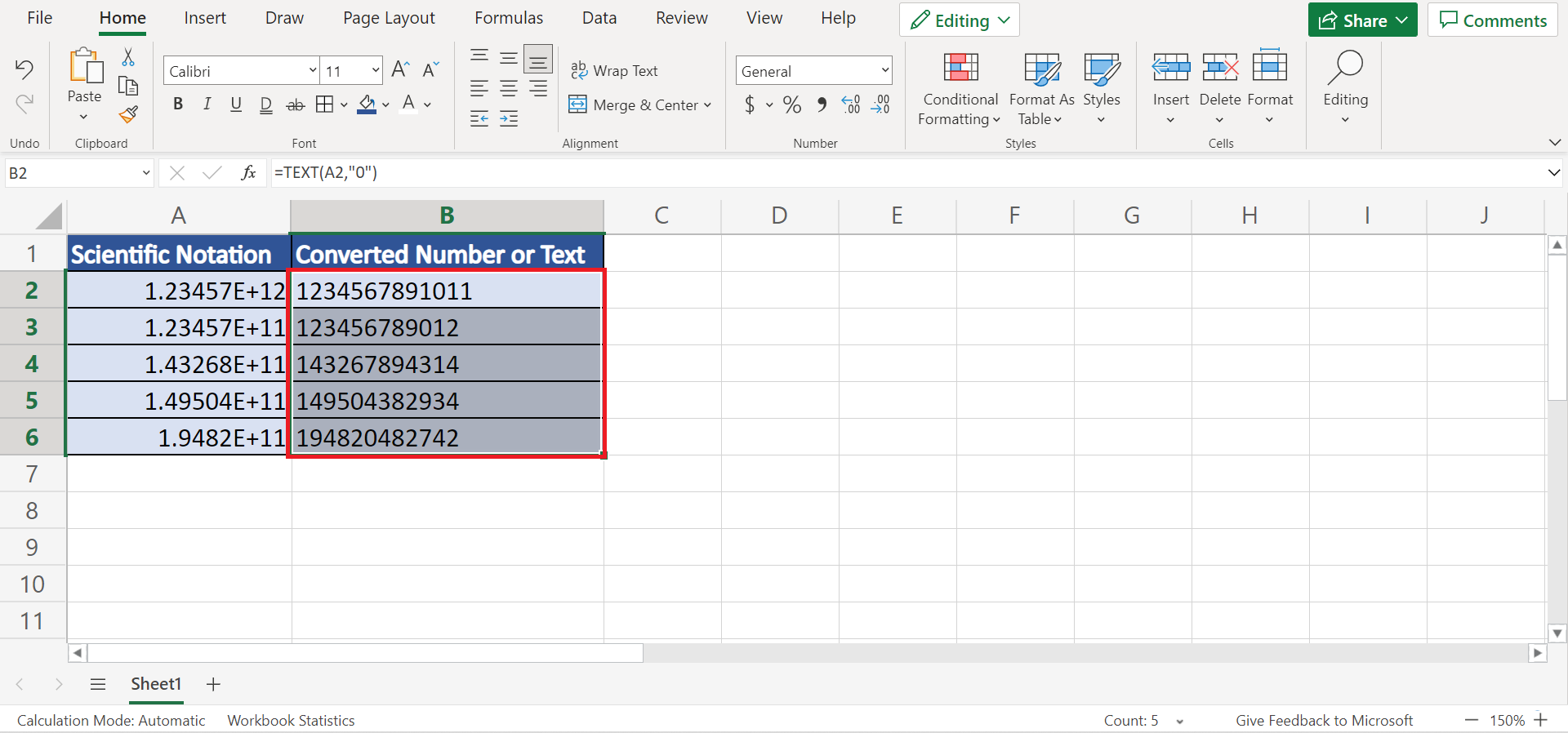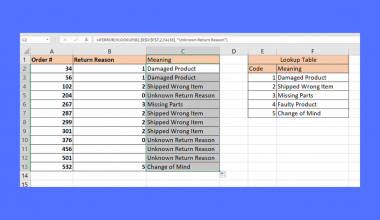# How to Convert Scientific Notation to Number or Text in Excel

This guide will explain how to convert scientific notation to numbers or text in Excel.

Excel is a handy tool that makes work easier for us. But, one thing Excel does which can be annoying is that it converts numbers with more than 11 digits into a scientific notation.

So the scientific notation format displays the number in exponential notation. And this can be an inconvenience when you want to show the entire length of the numbers.

But, worry not. There are several methods we can try to fix this issue and display the entirety of the numbers. So we can simply change the format of the numbers, or we can also extend the size of the cells to have more space to display the numbers. And this would convert the scientific notation to numbers.

Additionally, we can also convert them to text by utilizing the `TRIM` function, the `UPPER` function, and the `TEXT` function.

Let’s take a sample scenario.

Suppose you are inputting long digit numbers. And you start noticing that Excel converts them into the scientific notation format. But, you want the entire number to be displayed. What methods can you use?

Great! Let’s first start learning how to convert scientific notation to numbers in Excel using two easy and quick methods.

## Converting Scientific Notation to Number in Excel by Changing the Number Format

The first method we will use to convert scientific notation to numbers is simply changing the number format. So we can simply go to the Number Format and select a type of format to apply to the numbers. Then, we will be able to display the full length of the numbers.

To do this method, follow the steps below:

1. Firstly, we must select the cells we want to convert. Then, right-click and select Number Format.2. Secondly, the Number Format window will appear. So go to the Custom category and select “0” under the Type dropdown menu. Lastly, click OK to apply the changes.3. And tada! We have successfully converted the scientific notation to numbers in Excel.## Converting Scientific Notation to Number in Excel by Resizing the Cell

Another method we can use to convert scientific notation to numbers is simply adjusting the cells’ size. So there are times when the cell size is just not enough to display the entire number. And we can fix this by simply adjusting the size to fit the number.

1. Firstly, hover the cursor to the column containing your data or wherever you want to input the numbers. Then, simply click and drag the column line to the right to resize its column length.2. And tada! Now you can type in more than 11 digit numbers without having Excel convert them to scientific notation.Awesome! We have learned two easy methods of how to convert scientific notation to numbers in Excel. And before we start learning how to convert them to text, let’s first understand the syntax of the functions we will be using.

## The Anatomy of the TRIM Function

The syntax or the way we write the `TRIM` function is as follows:

`=TRIM(text)`

Let’s dissect this formula and understand what each term means:

• = is how we activate any function in Excel.
• TRIM() refers to our `TRIM` function. And this function will remove any spaces from a text string except for the single spaces between words.
• text is the only argument required for this function. So it refers to the text which you want to remove spaces from.

## The Anatomy of the UPPER Function

The syntax or the way we write the `UPPER` function is as follows:

`=UPPER(text)`

Let’s dissect this formula and understand what each term means:

• = the equal sign is how we start any function in Excel.
• UPPER() is our `UPPER` function. And this function will convert the selected cell or text string to uppercase letters.
• text is a required argument. So it refers to the text you want to convert to uppercase. And this can be a cell reference or text string.

Now let’s dive into the process of how to convert scientific notation to numbers or text in Excel. In this case, we will now focus on three ways to convert them to text using functions.

## Converting Scientific Notation to Text in Excel using the UPPER function

In this method, we will be using the `UPPER` function to convert the scientific notation to text in Excel.

To do this method, let’s learn the step-by-step process below:

1. Firstly, input the `UPPER` function and select the cell containing the scientific notation we will be converting. So the entire formula would be “UPPER(A2)”. Then, press the Enter key to return the results.2. Secondly, copy the formula by dragging it down to the rest of the rows.3. And tada! We have successfully converted scientific notation to numbers or text in Excel.## Converting Scientific Notation to Text in Excel using the TRIM function

So this time, we will use the `TRIM` function to convert our scientific notation to text in Excel. Simply follow the steps below to apply this method to your work.

1. Firstly, we will type in the `TRIM` function and select the cell containing the scientific notation. Then, the entire formula would be “TRIM(A2)”. Lastly, press the ENTER key to get the result.2. Secondly, we will drag down the formula to apply to the rest of the rows.3. And tada! We have converted the scientific notation using the `TRIM` function in Excel.## Converting Scientific Notation to Text in Excel using the TEXT function

Lastly, we can convert scientific notation to text in Excel using the `TEXT` function.

So let’s learn how to do this by following the steps below:

1. Firstly, we will input the `TEXT` function and select cell A2, which contains the scientific notation. Next, we will type in the number format, which is 0. So the entire formula would be “TEXT(A2,”0”)”. Then, press the ENTER key to convert the number.2. Again, we can drag down the formula to copy it to the rest of the rows.3. And tada! We have converted the scientific notation to text in Excel.And that’s pretty much it! We have discussed five easy and simple methods of how to convert scientific notation to numbers or text in Excel.  Now you have several solutions whenever you encounter this issue.

Are you interested in learning more about what Excel can do? You can now use the `TRIM` function and `UPPER` function and the various other Microsoft Excel formulas available to create great worksheets that work for you. Make sure to subscribe to our newsletter to be the first to know about the latest guides and tutorials from us.Our goal this year is to create lots of rich, bite-sized tutorials for Google Sheets users like you. If you liked this one, you'll love what we are working on! Readers receive ✨ early access ✨ to new content.

##### You May Also Like## How to Convert Multiple Rows to Columns and Rows in Excel

This guide will show you how you can convert multiple rows to columns and columns to rows in…## How to Calculate Mean Squared Error in Excel

This guide will discuss how to calculate mean squared error in Excel using the AVERAGE function. The rules…## How to Use SUBTOTAL with COUNTIF in Excel

This guide will discuss how to use SUBTOTAL with COUNTIF in Excel. The rules for using the COUNTIF…## How to Use ISERROR with VLOOKUP in Excel

This guide will explain how you can use the ISERROR function in Excel to catch and handle errors…